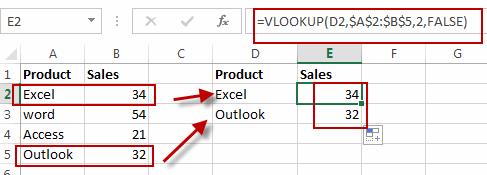# How to Compare Two Columns and Return Values in Third Column in Excel

This post will guide you how to compare two columns using VLOOKUP and return values from the third column in Excel. How do I match two columns in Excel and return a value in third column with a formula.

## Compare Two Columns and Return value in Third Column

Assuming that you have two list of data in your worksheet, the first data (A1:B5) contain product names and its sale values. and the second data table (D1:E3) contain only product names, and you want to compare product name columns for these two data, and if matched, then return the corresponding sale value from the first data based on the product in the second data.

You can use a formula based on the VLOOKUP function. Like this:

`=VLOOKUP(D2,\$A\$2:\$B\$5,2,FALSE)`

Type this formula into a blank cell E2 and press Enter key on your keyboard. and drag the AutoFill Handle down to cell E3 to apply this formula.### Related Functions

• Excel VLOOKUP function
The Excel VLOOKUP function lookup a value in the first column of the table and return the value in the same row based on index_num position.The syntax of the VLOOKUP function is as below:= VLOOKUP (lookup_value, table_array, column_index_num,[range_lookup])….